## Friday, July 15, 2011

### Weekly 3LB Update 7/15/11

Weekly 3LB update (week ending 7/15/11)
a new high or low will change the reversal price and the midpointSPX
-no change (above mid)
trend=no
direction=down (2 bars)
low= 1268.45
rev= 1363.61; mid= 1316.03

monthly info -no change (above mid)
trend=up
high= 1363.61
rev= 1257.64; mid= 1310.63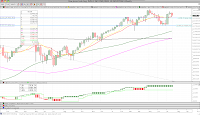Dow Jones
-no change (above mid)
trend=no
direction=up (2 bars)
high= 12810.54
rev= 11858.52; mid= 12334.53

monthly info -no change (above mid)
trend=up
high= 12810.54
rev= 11891.93; mid= 12351.24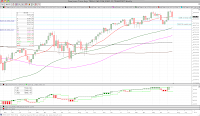Dow Jones Transportation Avg
-no change (above mid)
trend=no
direction=up (2 bars)
high= 5548.66
rev= 5060.59; mid= 5304.63

monthly info -no change (above mid)
trend=up
high= 5514.87
rev= 4855.78; mid= 5185.33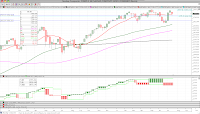Nasdaq Composite
-no change (above mid)
trend=no
direction=down (1 bar)
low= 2616.48
rev= 2873.54; mid= 2745.01

monthly info -no change (above mid)
trend=up
high= 2873.54
rev= 2643.67; mid= 2763.21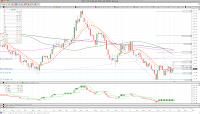DXY
-no change (above mid)
trend=no
direction=up (1 bar)
high= 75.76
rev= 72.93; mid= 74.35

monthly info -no change (below mid)
trend=down
low= 72.93
rev= 77.27; mid= 75.10Gold
new high 1590.10
trend=up
high= 1590.10
rev= 1486.90; mid= 1538.50

monthly info -no change (above mid)
trend=up
high= 1557.30
rev= 1389.70; mid= 1473.50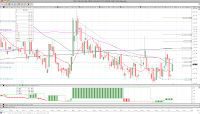VIX
-no change (above mid)
trend=up
high= 21.85
rev= 15.32; mid= 18.59

monthly info -no change (below mid)
trend=no; direction=down (1 bar)
low= 14.75
rev= 34.54; mid= 24.65WTI Crude Oil
-no change (below mid)
trend=down
low= 91.16
rev= 114.31; mid= 102.74

monthly info -no change (below mid)
trend=up
high= 114.45
rev= 80.85; mid= 97.65Sugar #11
-no change (above mid)
trend=no
direction=up (1 bar)
high= 29.36
rev= 18.45; mid= 23.91

monthly info -no change (above mid)
trend=up
high= 29.78
rev= 19.29; mid= 24.5430 Year Yield
-no change (below mid)
trend=no
direction=up (1 bar)
high= 44.02
rev= 41.74; mid= 42.88

monthly info -no change (above mid)
trend=up; direction=up (2 bars)
high= 47.15
rev= 26.91; mid= 37.0310 Year Yield
-no change (below mid)
trend=no
direction=up (1 bar)
high= 31.97
rev= 28.71; mid= 30.34

monthly info -no change (above mid)
trend=no; direction=up (1 bar)
high= 38.43
rev= 22.44; mid= 30.44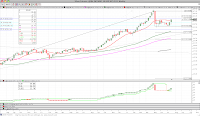Silver
WEEKLY CONFIRMATION new high 39.08
trend=no
direction=up (2 bars)
high= 39.08
rev= 33.70; mid= 36.39

monthly info -no change (below mid)
trend=up
high= 48.60
rev= 30.96; mid= 39.78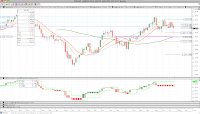EURUSD
-no change (below mid)
trend=no
direction=down (2 bars)
low= 1.4100
rev= 1.4820; mid= 1.4460

monthly info -no change (above mid)
trend=up
high= 1.4820
rev= 1.3605; mid= 1.4213GS (Goldman Sachs)
new low 130.20
trend=down
low= 130.16
rev= 141.46; mid= 135.81

monthly info -no change (above mid)
trend=no; direction=up (2 bars)
high= 184.35
rev= 78.99; mid= 131.67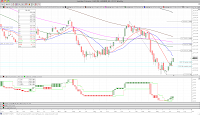LUMBER
new high 258.00
trend=up
high= 258.00
rev= 236.00; mid= 247.00

monthly info -no change (below mid)
trend=no; direction=down (2 barS)
low= 243.50
rev= 357.20; mid= 300.35BALTIC DRY INDEX
-no change (above mid)
trend=no
direction=up (2 bars)
high= 1585.00
rev= 1043.00; mid= 1314.00

monthly info - no change (below mid)
trend=down
low= 715.00
rev= 9370.00; mid= 5042.50XLF (SPDR Financial)
-no change (below mid)
trend=down
low= 14.76
rev= 15.68; mid= 15.22

monthly info -no change (above mid)
trend=no; direction=up (2 bars)
high= 16.85
rev= 13.55; mid= 15.20AUDJPY
-no change (below mid)
trend=up
high= 0.8864
rev= 0.8241; mid= 0.8553

monthly info -no change (above mid)
trend=up
high= 0.8845
rev= 0.7114; mid= 0.7980YEN
new high 1.26530
trend=up
high= 1.26530
rev= 1.24180; mid= 1.25360

monthly info -no change (above mid)
trend=up
high= 1.24330
rev= 1.15910; mid= 1.20120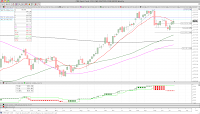CRB
-no change (below mid)
trend=down
low= 329.89
rev= 368.70; mid= 349.30

monthly info MONTHLY REVERSAL new low 338.05
trend=up
low= 338.05
rev= 370.56; mid= 354.31CAT (Caterpillar)
-no change (above mid)
trend=no
direction=up (2 bars)
high= 110.41
rev= 95.95; mid= 103.18

monthly info -no change (above mid)
trend=up
high= 115.41
rev= 97.01; mid= 106.21AA (Alcoa)
-no change (below mid)
trend=no
direction=up (2 bars)
high= 16.38
rev= 14.72; mid= 15.55

monthly info -no change (above mid)
trend=up
high= 17.66
rev= 11.64; mid= 14.65

BIDU (Baidu)
-no change (above mid)
trend=no
direction=up (1 bar)
high= 147.78
rev= 117.68; mid= 132.73

JPM (JP Morgan Chase)
-no change (below mid)
trend=no
direction=up (1 bar)
high= 41.58
rev= 39.49; mid= 40.54

BAC (Bank of America)
new low 10.00
trend=down
low= 10.00
rev= 10.80; mid= 10.40

WFC (Wells Fargo)
-no change (below mid)
trend=no
direction=up (1 bar)
high= 28.67
rev= 26.27; mid= 27.47

AXP (American Express)
-no change (above mid)
trend=up
high= 53.07
rev= 50.20; mid= 51.64

AMZN (Amazon)
-no change (above mid)
trend=up
high= 218.28
rev= 197.60; mid= 207.94

M (Macy's)
-no change (above mid)
trend=up
high= 30.42
rev= 28.63; mid= 29.53

BKX (KBW Bank Index)
new low 46.29
trend=down
low= 46.29
rev= 47.69; mid= 46.99

Powershares USD Bull (UUP)
-no change (above mid)
trend=no
direction=up (1 bar)
high= 21.69
rev= 20.95; mid= 21.32

TLT (iShares Barclays 20+ Year Treas Bond)
-no change (above mid)
trend=no
direction=down (1 bar)
low= 93.63
rev= 97.14; mid= 95.39

AAPL (Apple Computer)
new high 364.92
trend=up
high= 364.92
rev= 325.90; mid= 345.41

V (Visa)
-no change (above mid)
trend=no
direction=up (2 bars)
high= 89.73
rev= 66.90; mid= 78.32

XRT (SPDR Retail ETF)
-no change (above mid)
trend=no
direction=up (2 bars)
high= 55.91
rev= 49.48; mid= 52.70

MUB (iShares S&P National AMT-Fre)
-no change (above mid)
trend=no
direction=down (2 bars)
low= 102.87
rev= 103.88; mid= 103.38

IQI (Morgan Stanley Quality Muni)
-no change (above mid)
trend=no
direction=up (1 bar)
high= 12.64
rev= 11.19; mid= 11.92

JNK (SPDR Barclays Capital HY)
-no change (below mid)
trend=down
low= 39.38
rev= 40.88; mid= 40.13

COPPER
-no change (above mid)
trend=no
direction=down (2 bars)
low= 3.976
rev= 4.615; mid= 4.296

EURCHF
new low 1.1477
trend=down
low= 1.1477
rev= 1.2126; mid= 1.1802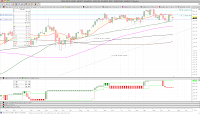EEM (MSCI Emerging Markets)
-no change (below mid)
trend=down
low= 45.50
rev= 50.04; mid= 47.77

monthly info -no change (below mid)
trend=up
high= 50.00
rev= 46.12; mid= 48.05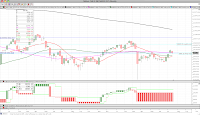NIKKEI
-no change (above mid)
trend=down
low= 9206.75
rev= 10605.65; mid= 9906.20

monthly info -no change (below mid)
trend=no; direction=down (1 bar)
low= 8824.06
rev= 11089.94; mid= 9957.00HANG SENG
-no change (below mid)
trend=no
direction=down (2 bars)
low= 21695.26
rev= 24876.82; mid= 23286.04

monthly info MONTHLY REVERSAL new low 22398.10
trend=no; direction=down (1 bar)
low= 22398.10
rev= 23720.81; mid= 23059.46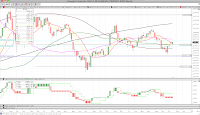SHANGHAI Composite
-no change (above mid)
trend=down
low= 2642.82
rev= 2858.46; mid= 2750.64

monthly info -no change (above mid)
trend=no; direction=up (2 bars)
high= 3412.06
rev= 1728.79; mid= 2570.43KOSPI
-no change (above mid)
trend=no
direction=up (2 bars)
high= 2139.14
rev= 2031.93; mid= 2085.54

monthly info -no change (below mid)
trend=up
high= 2192.36
rev= 2051.00; mid= 2121.68Athens
WEEKLY REVERSAL new low 1176.88
trend=no
direction=down (1 bar)
low= 1176.88
rev= 1308.48; mid= 1242.68

monthly info MONTHLY CONFIRMATION new low 1279.06
trend=no; direction=down (2 bars)
low= 1279.06
rev= 1593.30; mid= 1436.18

## Sunday, July 10, 2011

### Weekly 3LB Update 7/8/11

Weekly 3LB update (week ending 7/8/11)
a new high or low will change the reversal price and the midpointSPX
-no change (above mid)
trend=no
direction=down (2 bars)
low= 1268.45
rev= 1363.61; mid= 1316.03

monthly info -no change (above mid)
trend=up
high= 1363.61
rev= 1257.64; mid= 1310.63Dow Jones
-no change (above mid)
trend=no
direction=up (2 bars)
high= 12810.54
rev= 11858.52; mid= 12334.53

monthly info -no change (above mid)
trend=up
high= 12810.54
rev= 11891.93; mid= 12351.24Dow Jones Transportation Avg
WEEKLY CONFIRMATION new high 5548.66
trend=no
direction=up (2 bars)
high= 5548.66
rev= 5060.59; mid= 5304.63

monthly info -no change (above mid)
trend=up
high= 5514.87
rev= 4855.78; mid= 5185.33Nasdaq Composite
-no change (above mid)
trend=no
direction=down (1 bar)
low= 2616.48
rev= 2873.54; mid= 2745.01

monthly info -no change (above mid)
trend=up
high= 2873.54
rev= 2643.67; mid= 2763.21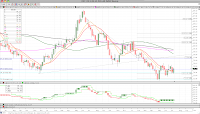DXY
-no change (above mid)
trend=no
direction=up (1 bar)
high= 75.76
rev= 72.93; mid= 74.35

monthly info -no change (below mid)
trend=down
low= 72.93
rev= 77.27; mid= 75.10Gold
-no change (above mid)
trend=up
high= 1557.30
rev= 1475.00; mid= 1516.15

monthly info -no change (above mid)
trend=up
high= 1557.30
rev= 1389.70; mid= 1473.50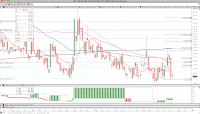VIX
-no change (below mid)
trend=up
high= 21.85
rev= 15.32; mid= 18.59

monthly info -no change (below mid)
trend=no; direction=down (1 bar)
low= 14.75
rev= 34.54; mid= 24.65WTI Crude Oil
-no change (below mid)
trend=down
low= 91.16
rev= 114.31; mid= 102.74

monthly info -no change (below mid)
trend=up
high= 114.45
rev= 80.85; mid= 97.65Sugar #11
WEEKLY REVERSAL new high 29.36
trend=no
direction=up (1 bar)
high= 29.36
rev= 18.45; mid= 23.91

monthly info -no change (above mid)
trend=up
high= 29.78
rev= 19.29; mid= 24.5430 Year Yield
-no change (below mid)
trend=no
direction=up (1 bar)
high= 44.02
rev= 41.74; mid= 42.88

monthly info -no change (above mid)
trend=up; direction=up (2 bars)
high= 47.15
rev= 26.91; mid= 37.0310 Year Yield
-no change (above mid)
trend=no
direction=up (1 bar)
high= 31.97
rev= 28.71; mid= 30.34

monthly info -no change (above mid)
trend=no; direction=up (1 bar)
high= 38.43
rev= 22.44; mid= 30.44Silver
WEEKLY REVERSAL new high 36.54
trend=no
direction=up (1 bar)
high= 36.54
rev= 33.70; mid= 35.12

monthly info -no change (below mid)
trend=up
high= 48.60
rev= 30.96; mid= 39.78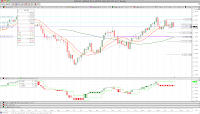EURUSD
-no change (below mid)
trend=no
direction=down (2 bars)
low= 1.4100
rev= 1.4820; mid= 1.4460

monthly info -no change (above mid)
trend=up
high= 1.4820
rev= 1.3605; mid= 1.4213GS (Goldman Sachs)
-no change (below mid)
trend=down
low= 130.91
rev= 150.10; mid= 140.51

monthly info -no change (above mid)
trend=no; direction=up (2 bars)
high= 184.35
rev= 78.99; mid= 131.67LUMBER
new high 248.80
trend=up
high= 248.80
rev= 226.50; mid= 237.65

monthly info -no change (below mid)
trend=no; direction=down (2 barS)
low= 243.50
rev= 357.20; mid= 300.35BALTIC DRY INDEX
-no change (above mid)
trend=no
direction=up (2 bars)
high= 1585.00
rev= 1043.00; mid= 1314.00

monthly info - no change (below mid)
trend=down
low= 715.00
rev= 9370.00; mid= 5042.50XLF (SPDR Financial)
-no change (above mid)
trend=down
low= 14.76
rev= 15.68; mid= 15.22

monthly info -no change (above mid)
trend=no; direction=up (2 bars)
high= 16.85
rev= 13.55; mid= 15.20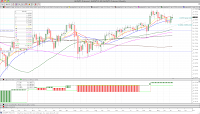AUDJPY
-no change (above mid)
trend=up
high= 0.8864
rev= 0.8241; mid= 0.8553

monthly info -no change (above mid)
trend=up
high= 0.8845
rev= 0.7114; mid= 0.7980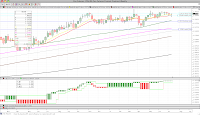YEN
-no change (below mid)
trend=up
high= 1.25020
rev= 1.23200; mid= 1.24110

monthly info -no change (above mid)
trend=up
high= 1.24330
rev= 1.15910; mid= 1.20120CRB
-no change (below mid)
trend=down
low= 329.89
rev= 368.70; mid= 349.30

monthly info MONTHLY REVERSAL new low 338.05
trend=up
low= 338.05
rev= 370.56; mid= 354.31CAT (Caterpillar)
WEEKLY CONFIRMATION new high 110.41
trend=no
direction=up (2 bars)
high= 110.41
rev= 95.95; mid= 103.18

monthly info -no change (above mid)
trend=up
high= 115.41
rev= 97.01; mid= 106.21AA (Alcoa)
WEEKLY REVERSAL new high 16.38
trend=no
direction=up (2 bars)
high= 16.38
rev= 14.72; mid= 15.55

monthly info -no change (above mid)
trend=up
high= 17.66
rev= 11.64; mid= 14.65

BIDU (Baidu)
WEEKLY REVERSAL new high 147.78
trend=no
direction=up (1 bar)
high= 147.78
rev= 117.68; mid= 132.73

JPM (JP Morgan Chase)
-no change (above mid)
trend=no
direction=up (1 bar)
high= 41.58
rev= 39.49; mid= 40.54

BAC (Bank of America)
-no change (below mid)
trend=down
low= 10.52
rev= 11.28; mid= 10.90

WFC (Wells Fargo)
-no change (above mid)
trend=no
direction=up (1 bar)
high= 28.67
rev= 26.27; mid= 27.47

AXP (American Express)
new high 53.07
trend=up
high= 53.07
rev= 50.20; mid= 51.64

AMZN (Amazon)
new high 218.28
trend=up
high= 218.28
rev= 197.60; mid= 207.94

M (Macy's)
new high 30.42
trend=up
high= 30.42
rev= 28.63; mid= 29.53

BKX (KBW Bank Index)
-no change (below mid)
trend=down
low= 46.32
rev= 49.51; mid= 47.92

Powershares USD Bull (UUP)
-no change (above mid)
trend=no
direction=up (1 bar)
high= 21.69
rev= 20.95; mid= 21.32

TLT (iShares Barclays 20+ Year Treas Bond)
-no change (below mid)
trend=no
direction=down (1 bar)
low= 93.63
rev= 97.14; mid= 95.39

AAPL (Apple Computer)
WEEKLY CONFIRMATION new high 359.71
trend=no
direction=up (2 bars)
high= 359.71
rev= 320.26; mid= 339.99

V (Visa)
WEEKLY CONFIRMATION new high 89.73
trend=no
direction=up (2 bars)
high= 89.73
rev= 66.90; mid= 78.32

XRT (SPDR Retail ETF)
WEEKLY CONFIRMATION new high 55.91
trend=no
direction=up (2 bars)
high= 55.91
rev= 49.48; mid= 52.70

MUB (iShares S&P National AMT-Fre)
WEEKLY CONFIRMATION new low 102.87
trend=no
direction=down (2 bars)
low= 102.87
rev= 103.88; mid= 103.38

IQI (Morgan Stanley Quality Muni)
-no change (above mid)
trend=no
direction=up (1 bar)
high= 12.64
rev= 11.19; mid= 11.92

JNK (SPDR Barclays Capital HY)
-no change (above mid)
trend=down
low= 39.38
rev= 40.88; mid= 40.13

COPPER
-no change (above mid)
trend=no
direction=down (2 bars)
low= 3.976
rev= 4.615; mid= 4.296

EURCHF
-no change (below mid)
trend=down
low= 1.1844
rev= 1.2970; mid= 1.2407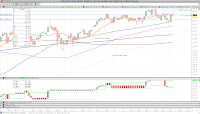EEM (MSCI Emerging Markets)
-no change (above mid)
trend=down
low= 45.50
rev= 50.04; mid= 47.77

monthly info -no change (below mid)
trend=up
high= 50.00
rev= 46.12; mid= 48.05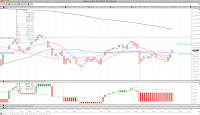NIKKEI
-no change (above mid)
trend=down
low= 9206.75
rev= 10605.65; mid= 9906.20

monthly info -no change (below mid)
trend=no; direction=down (1 bar)
low= 8824.06
rev= 11089.94; mid= 9957.00HANG SENG
-no change (below mid)
trend=no
direction=down (2 bars)
low= 21695.26
rev= 24876.82; mid= 23286.04

monthly info MONTHLY REVERSAL new low 22398.10
trend=no; direction=down (1 bar)
low= 22398.10
rev= 23720.81; mid= 23059.46SHANGHAI Composite
-no change (above mid)
trend=down
low= 2642.82
rev= 2858.46; mid= 2750.64

monthly info -no change (above mid)
trend=no; direction=up (2 bars)
high= 3412.06
rev= 1728.79; mid= 2570.43KOSPI
WEEKLY CONFIRMATION new high 2139.14
trend=no
direction=up (2 bars)
high= 2139.14
rev= 2031.93; mid= 2085.54

monthly info -no change (below mid)
trend=up
high= 2192.36
rev= 2051.00; mid= 2121.68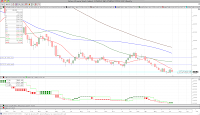Athens
-no change (below mid)
trend=no
direction=up (1 bar)
high= 1308.48
rev= 1232.60; mid= 1270.54

monthly info MONTHLY CONFIRMATION new low 1279.06
trend=no; direction=down (2 bars)
low= 1279.06
rev= 1593.30; mid= 1436.18